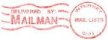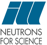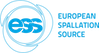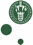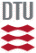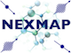McStas: Source_gen4 Component

# The `Source_gen4` Component

Circular/squared neutron source with flat or Maxwellian energy/wavelength spectrum (possibly spatially gaussian)

## Identification

• Author: Emmanuel Farhi, Kim Lefmann, modified to PSI use by Jonas Okkels Birk
• Origin: ILL/Risoe
• Date: Aug 27, 2001
• Version:(Unknown)
• Modification history:
• EF, Aug 27, 2001 ; can use Energy/wavelength and I1
• EF, Sep 18, 2001 ; corrected illumination bug. Add options
• EF, Oct 30, 2001 ; minor changes. mccompcurname replaced

## Description

```This routine is a neutron source (rectangular or circular), which aims at
a square target centered at the beam (in order to improve MC-acceptance
rate). The angular divergence is then given by the dimensions of the
target.
The neutron energy/wavelength is distributed between Emin=E0-dE and
Emax=E0+dE or Lmin=Lambda0-dLambda and Lmax=Lambda0+dLambda. The I1 may
be either arbitrary (I1=0), or specified in neutrons per steradian per
square cm per Angstrom. A Maxwellian spectra may be selected if you give
the source temperatures (up to 3). And a high energytail of the shape A/(lambda0-lambda) can also be
specified if you give an A. Finally, a file with the flux as a
function of the wavelength [lambda(AA) flux(n/s/cm^2/st/AA)] may be used
with the 'flux_file' parameter. Format is 2 columns free text.
Additionl distributions for the horizontal and vertical phase spaces
distributions (position-divergence) may be specified with the
'xdiv_file' and 'ydiv_file' parameters. Format is free text, requiring
a comment line '# xylimits: pos_min pos_max div_min div_max' to set
the axis of the distribution matrix. All these files may be generated using
standard monitors (better in McStas/PGPLOT format), e.g.:
Monitor_nD(options="auto lambda per cm2")
Monitor_nD(options="x hdiv, all auto")
Monitor_nD(options="y vdiv, all auto")
The source shape is defined by its radius, or can alternatively be squared
if you specify non-zero h and w parameters.
The beam is spatially uniform, but becomes gaussian if one of the source
dimensions (radius, h or w) is negative, or you set the gaussian flag.
Divergence profiles are triangular.
The source may have a thickness, which will broaden the default zero time
distribution.
For the Maxwellian spectra, the generated intensity is dPhi/dLambda (n/s/AA)
For flat spectra, the generated intensity is Phi (n/s).

Usage example:
Source_gen(h=0.1,w=0.1,Emin=1,Emax=3, I1=1e13, verbose=1, gaussian=1)
EXTEND
%{
t = rand0max(1e-3); // set time from 0 to 1 ms for TOF instruments.
%}

Some sources parameters:
PSI cold source T1= 296.16, I1=8.5E11, T2=40.68, I2=5.2E11
ILL VCS cold source T1=216.8,I1=1.24e+13,T2=33.9,I2=1.02e+13
(H1)         T3=16.7 ,I3=3.0423e+12
ILL HCS cold source T1=413.5,I1=10.22e12,T2=145.8,I2=3.44e13
(H5)         T3=40.1 ,I3=2.78e13
ILL Thermal tube    T1=683.7,I1=0.5874e+13,T2=257.7,I2=2.5099e+13
(H12)        T3=16.7 ,I3=1.0343e+12
ILL Hot source      T1=1695,I1=1.74e13,T2=708,I2=3.9e12

%VALIDATION
Feb 2005: output cross-checked for 3 Maxwellians against VITESS source
I(lambda), I(hor_div), I(vert_div) identical in shape and absolute values
Validated by: K. Lieutenant

```
WARNING: This is a contributed Component.

## Input parameters

Parameters in boldface are required; the others are optional.
Name Unit Description Default
flux_file str Name of a two columns [lambda flux] text file that contains the wavelength distribution of the flux in [1/(s*cm**2*st*AA)]. Comments (#) and further columns are ignored. Format is compatible with McStas/PGPLOT wavelength monitor files. When specified, temperature and intensity values are ignored. 0
xdiv_file str Name of the x-horiz. divergence distribution file, given as a free format text matrix, preceeded with a line '# xylimits: xmin xmax xdiv_min xdiv_max' 0
ydiv_file str Name of the y-vert. divergence distribution file, given as a free format text matrix, preceeded with a line '# xylimits: ymin ymax ydiv_min ydiv_max' 0
radius m Radius of circle in (x,y,0) plane where neutrons are generated. You may also use 'h' and 'w' for a square source 0.0
dist m Distance to target along z axis. 0
xw m Width(x) of target. If dist=0.0, xw = full horz. div in deg 0
yh m Height(y) of target. If dist=0.0, yh = full vert. div in deg 0
E0 meV Mean energy of neutrons. 0
dE meV Energy spread of neutrons, half width. 0
Lambda0 AA Mean wavelength of neutrons. 0
dLambda AA Wavelength spread of neutrons,half width 0
I1 1/(cm**2*st) Source flux per solid angle, area and Angstrom 0
h m Source y-height, then does not use radius parameter 0
w m Source x-width, then does not use radius parameter 0
gaussian 0/1 Use gaussian divergence beam, normal distributions 0
verbose 0/1 display info about the source. -1 unactivate source. 0
T1 K Temperature of the Maxwellian source, 0=none 0
flux_file_perAA 1 When true (1), indicates that flux file data is already per Angstroem. If false, file data is per wavelength bin. 0
flux_file_log 1 When true, will transform the flux table in log scale to improve the sampling. This may also cause 0
Lmin AA Minimum wavelength of neutrons 0
Lmax AA Maximum wavelength of neutrons 0
Emin meV Minimum energy of neutrons 0
Emax meV Maximum energy of neutrons 0
T2 K Second Maxwellian source Temperature, 0=none 0
I2 1/(cm**2*st) Second Maxwellian Source flux 0
T3 K Third Maxwellian source Temperature, 0=none 0
I3 1/(cm**2*st) Third Maxwellian Source flux 0
length m Source z-length, not anymore flat 0
phi_init degrees Angle abowe the x-z-plan that the source is amied at 0
theta_init degrees Angle from the z-axis in the x-z-plan that the source is amied at 0
HEtailA 1/(cm**2*st*AA) Amplitude of heigh energy tail 0
HEtailL0 AA Offset of heigh energy tail 0

## Output parameters

Name Unit Description Default
p_in
lambda0
lambda02
L2P
lambda0b
lambda02b
L2Pb
lambda0c
lambda02c
L2Pc
pTable
pTable_x
pTable_y